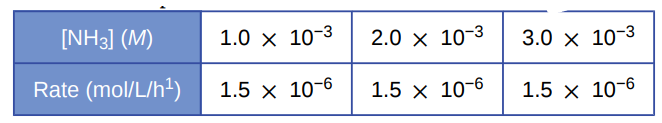# Problem: Under certain conditions the decomposition of ammonia on a metal surface gives the following data:Determine the rate equation, the rate constant, and the overall order for this reaction.

###### FREE Expert Solution

We’re being asked to determine the rate equation, the rate constant, and the overall order for the reaction based on the reaction and data given.

Recall that the rate law only focuses on the reactant concentrations and has a general form of:

k = rate constant
A & B = reactants
x & y = reactant orders

We will calculate the rate order first. Since we only have one reactant, we can use any of the data interval given:x = order

89% (48 ratings)###### Problem Details

Under certain conditions the decomposition of ammonia on a metal surface gives the following data:Determine the rate equation, the rate constant, and the overall order for this reaction.

Frequently Asked Questions

What scientific concept do you need to know in order to solve this problem?

Our tutors have indicated that to solve this problem you will need to apply the Rate Law concept. You can view video lessons to learn Rate Law. Or if you need more Rate Law practice, you can also practice Rate Law practice problems.

What textbook is this problem found in?

Our data indicates that this problem or a close variation was asked in Chemistry - OpenStax 2015th Edition. You can also practice Chemistry - OpenStax 2015th Edition practice problems.